Like   Tweet# It was everything I dreamed of

 table div table+table+table+table div table{width:100%;padding:0}table div table+table+table+table div table img{width:96.23%;padding:0;float:none}table div table+table+table+table div table td{width:100%;padding:0 1.88% 18px}/* styles */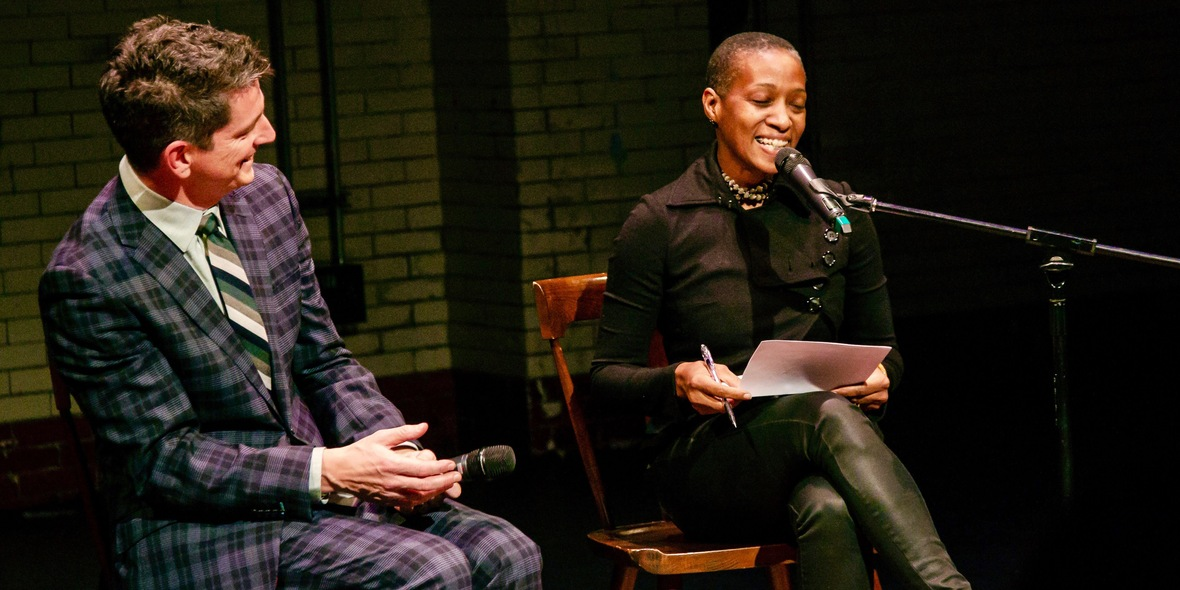/* styles */ Choreographer, writer, and all-around genius Tania Isaac joined me in a conversation about writing and making dances. Max Brown, costumer to the stars, outfitted me in that suit and tie. Turns out I’d never worn a suit that actually fit.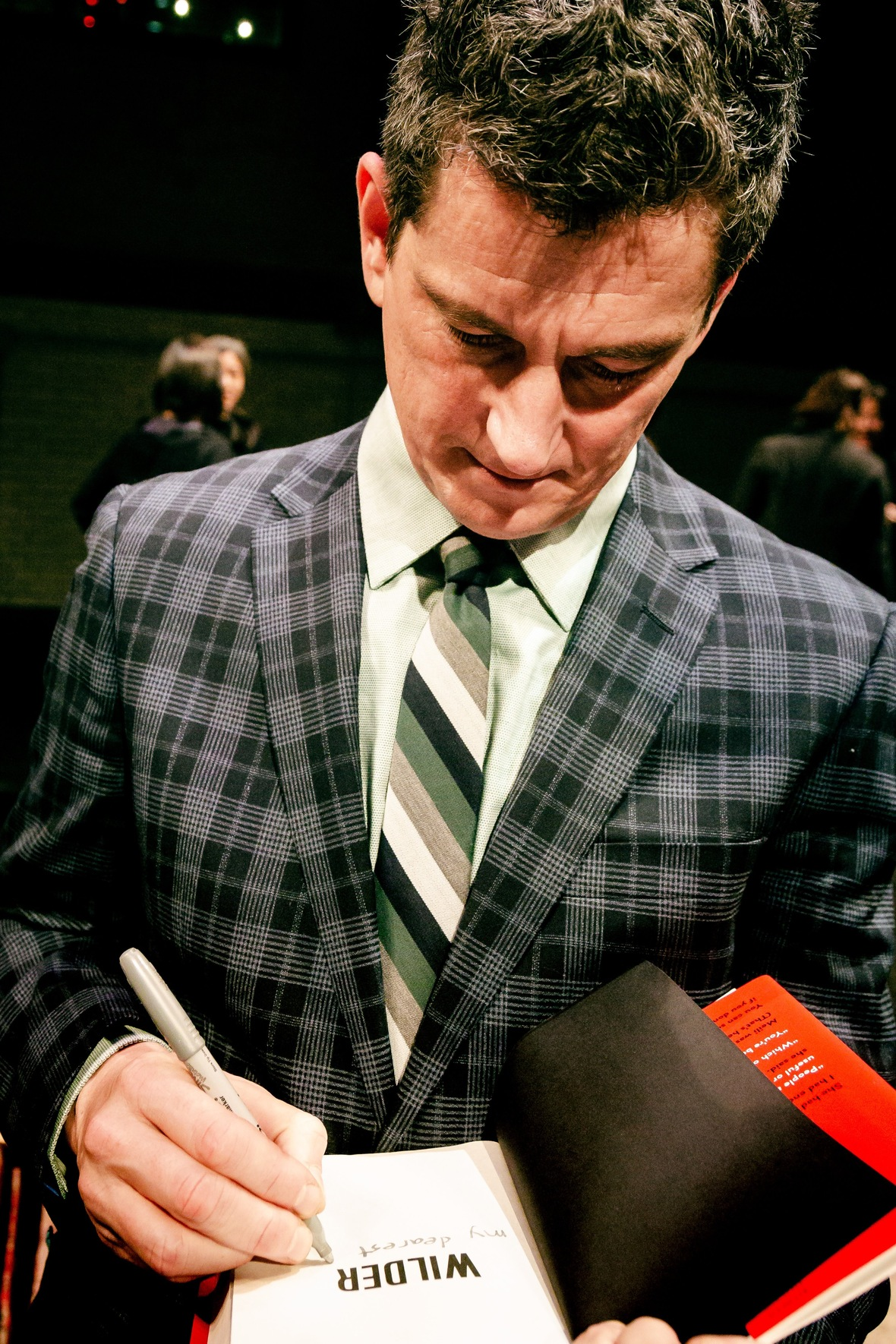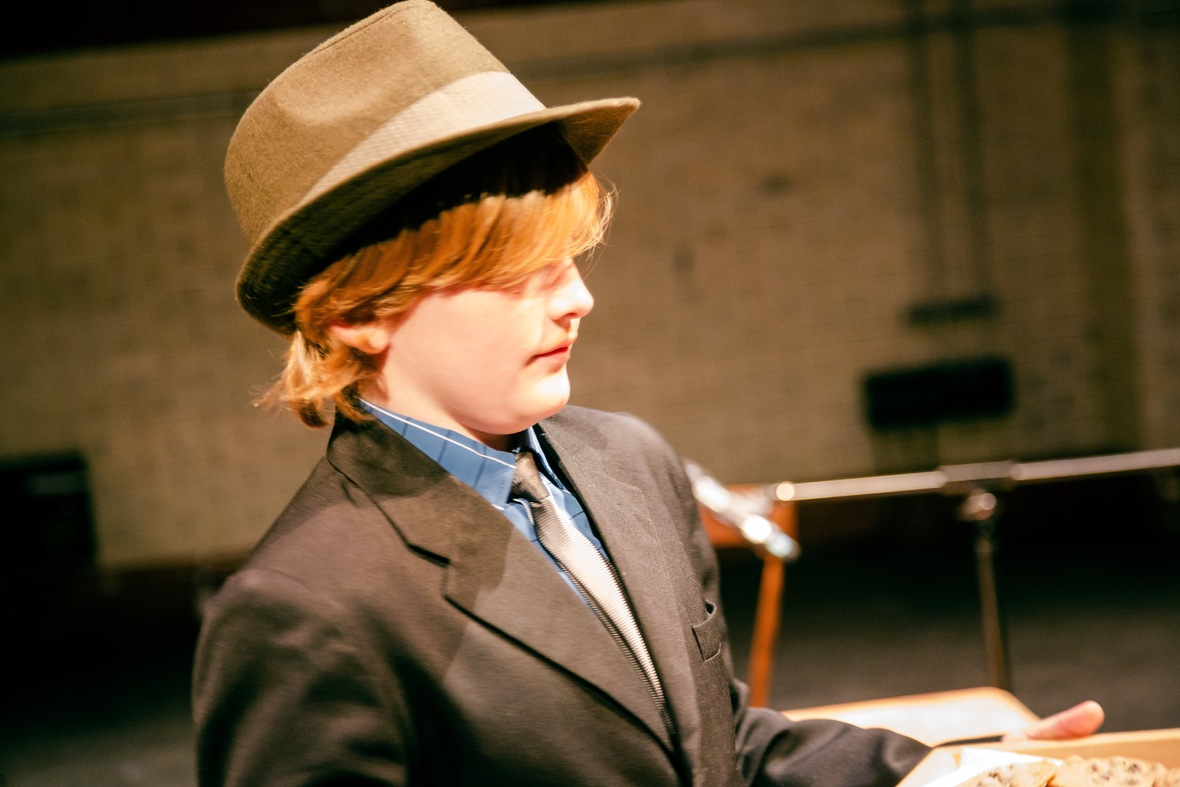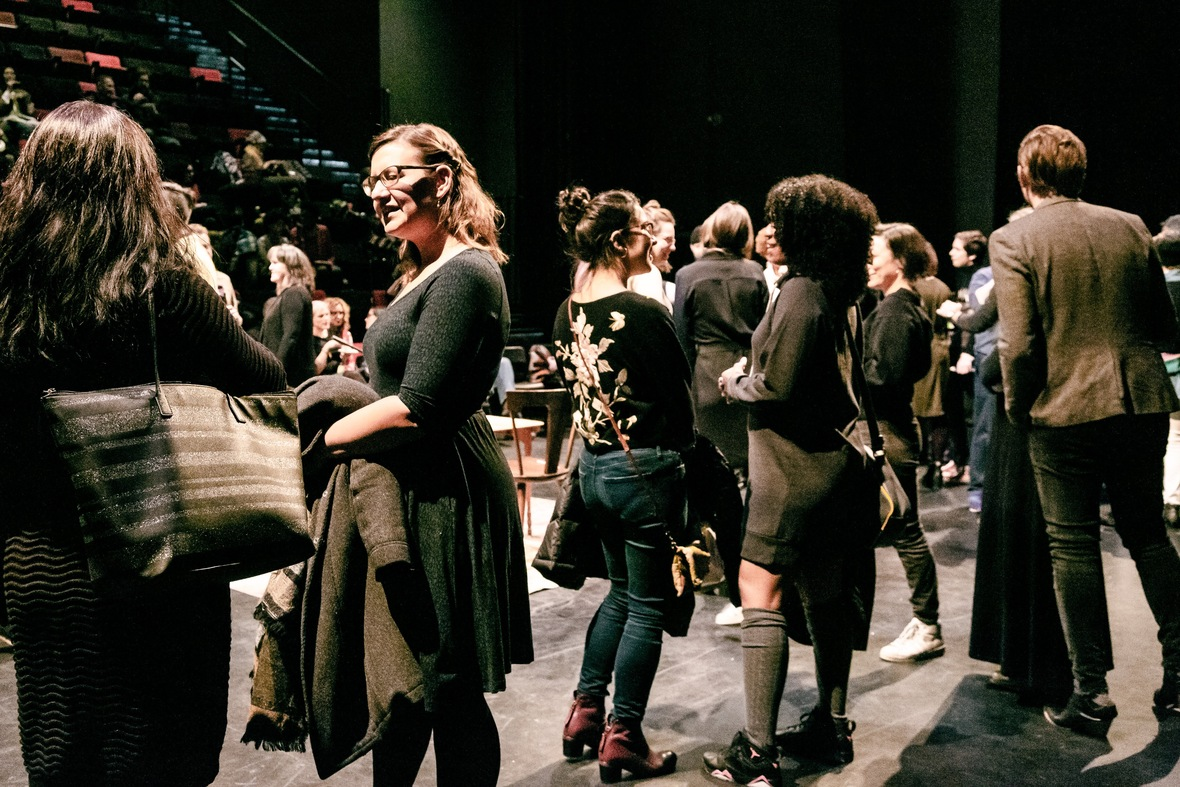/* styles */ Emmanuelle Delpech was the DJ, my kids helpd me make 150 chocolate chip cookies. And I gave a reading/talk called 13 Thoughts on Writing and Fighting. I talked about Wilder, about the long process of creation, and about masculinity and the stories we men tell ourselves. So many dear friends and colleagues came out. Writing, unlike dance, can be pretty damn isolating. This was the party I craved.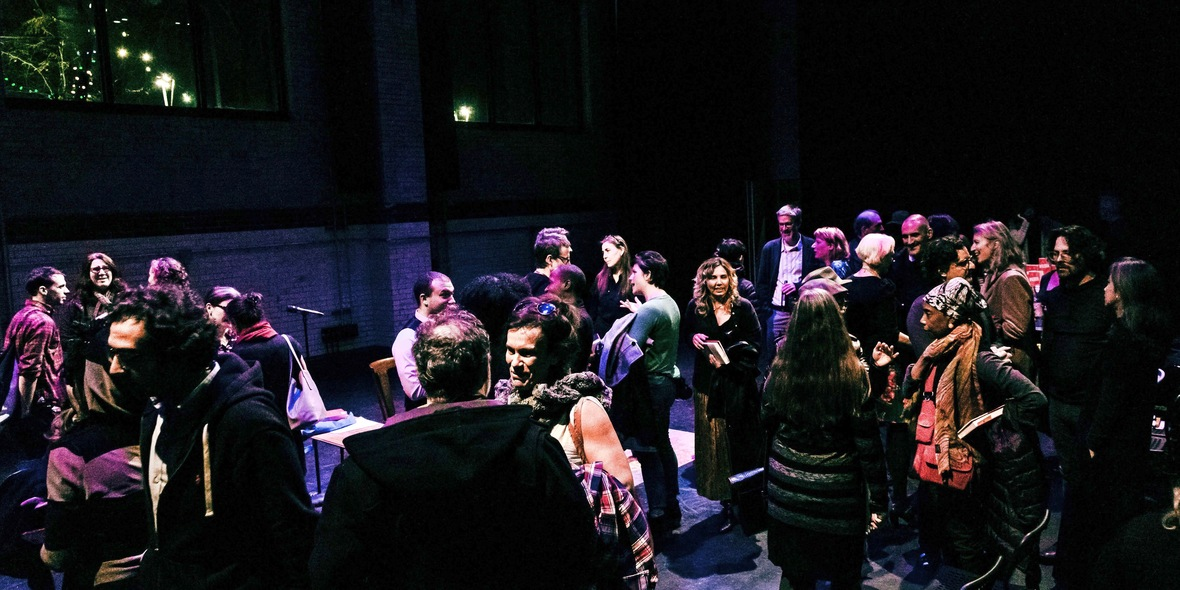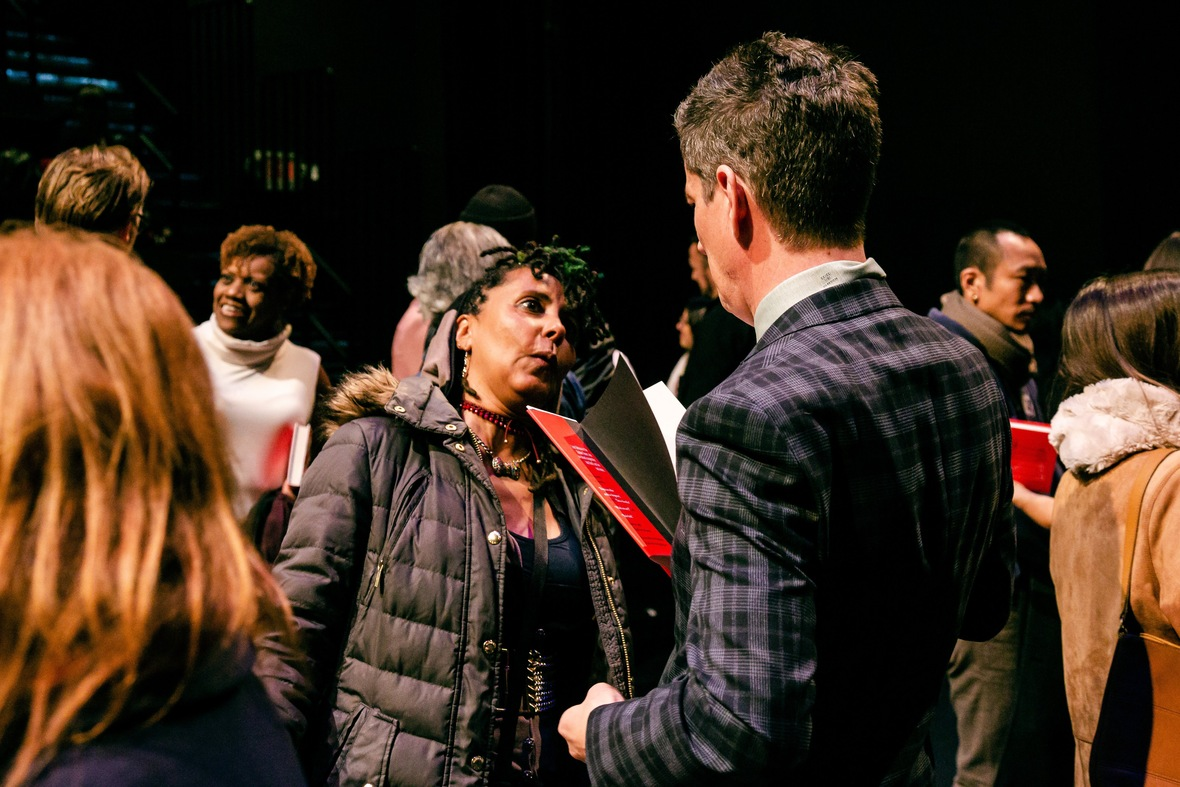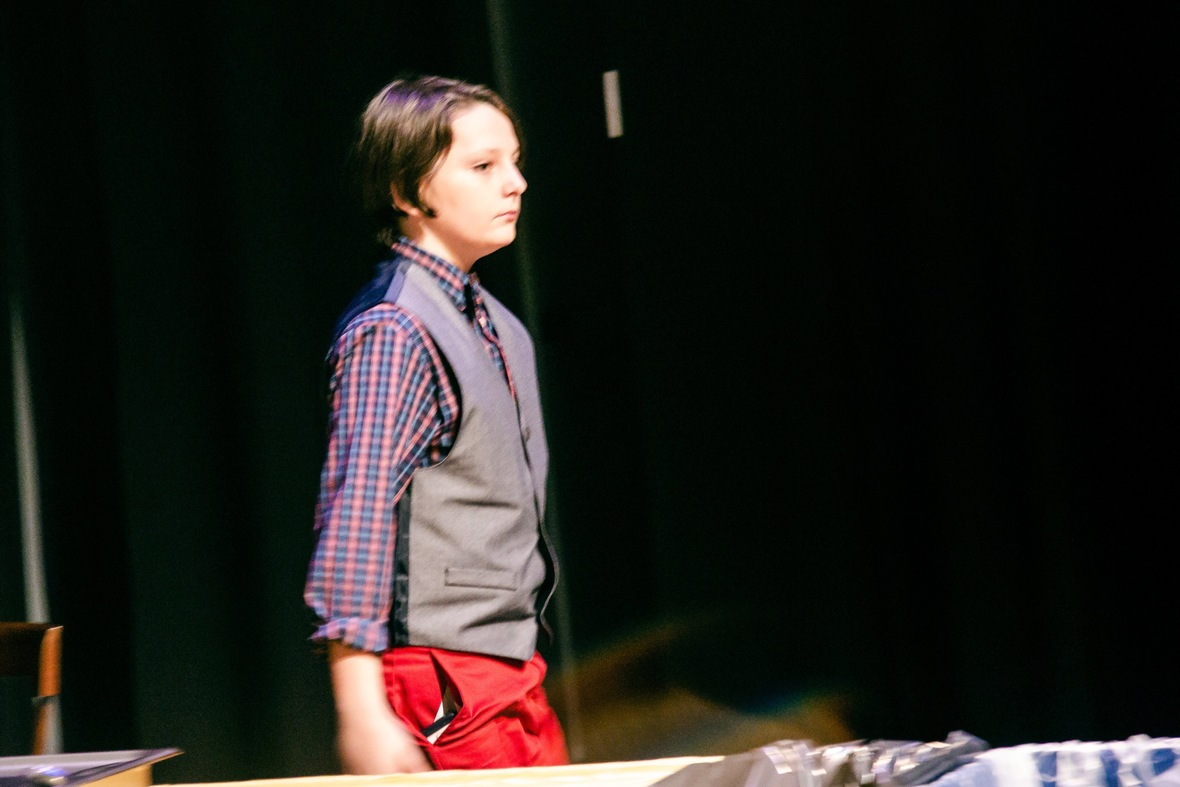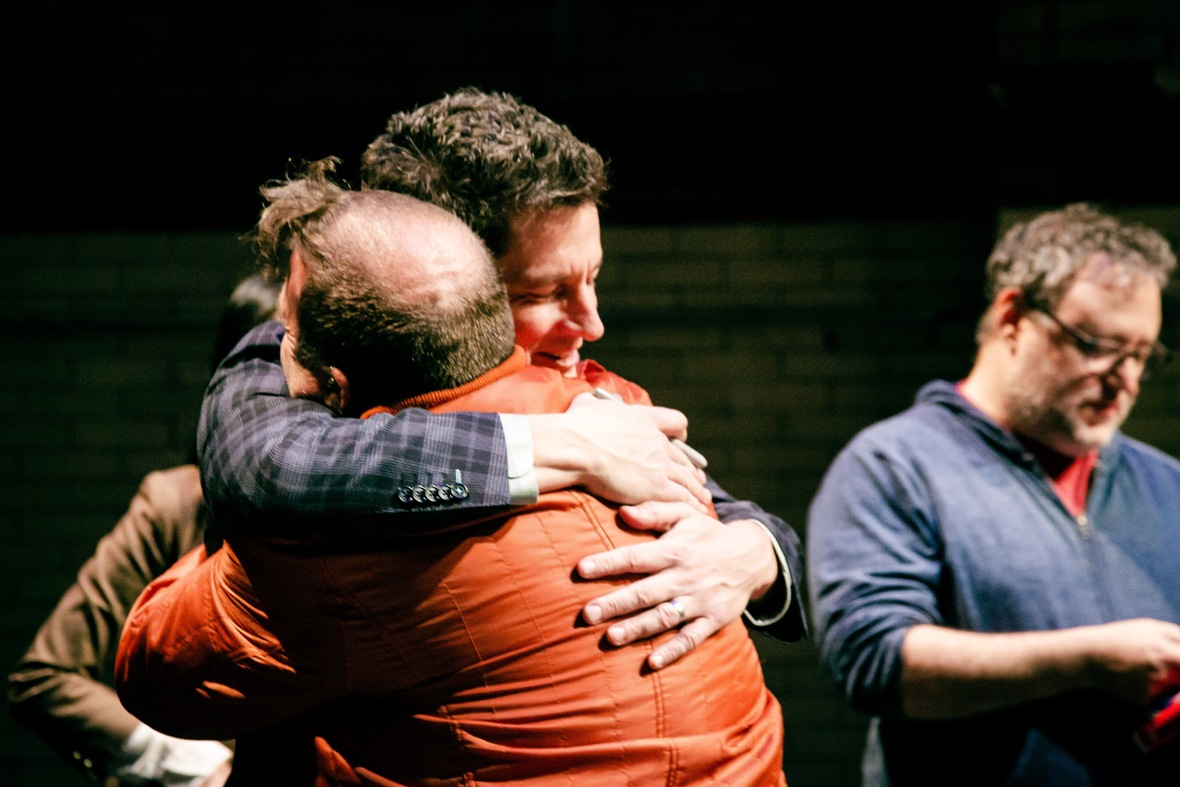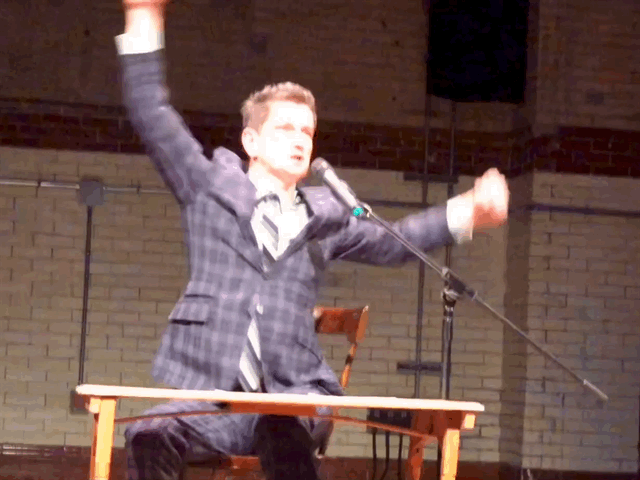Dance, author, dance!
 table div table+table+table+table+table+table+table+table+table+table+table+table div table{width:100%;padding:0}table div table+table+table+table+table+table+table+table+table+table+table+table div table img{width:96.23%;padding:0;float:none}table div table+table+table+table+table+table+table+table+table+table+table+table div table td{width:100%;padding:0 1.88% 18px}/* styles */table div table+table+table+table+table+table+table+table+table+table+table+table+table div table td,table.module-12{width:100%;padding:0}table div table+table+table+table+table+table+table+table+table+table+table+table+table div table{width:100%;float:none;margin-left:auto;margin-right:auto;padding:0}table div table+table+table+table+table+table+table+table+table+table+table+table+table div table a{border:0 none;text-decoration:none}table div table+table+table+table+table+table+table+table+table+table+table+table+table div table img{width:100%!important;border:0 none;text-decoration:none}/* styles */

# I'm doing events in NYC and Baltimore in the coming weeks

 /* styles */ NYC Teen Author Fest Friday March 29 I'm on a panel about complexity in YA relationships at the NYPL. And most thrillingly/embarrassingly, I will be part of a group of authors staging scenes from our novels at the Upper West Side Barnes and Noble. Info here. Baltimore’s CityLit Festival Saturday, April 27 I am on a panel with Susan Muaddi Darraj, who will introduce one of the first ever middle-grade series about an Arab-American girl, Tiffany D. Jackson, author of Monday's Not Coming, and Sharon G. Flake, who's celebrating the 20th anniversary of The Skin I'm In. More info soon. Rowan University Here’s a writing and performing challenge: I will be the commencement speaker at Rowan University’s College of Performing Arts on May 15. Twelve minutes to send these artists out into the world (and reassure their families). I’ll share whatever I come up with in a future email.
 table div table+table+table+table+table+table+table+table+table+table+table+table+table+table+table+table div table{width:100%;padding:0}table div table+table+table+table+table+table+table+table+table+table+table+table+table+table+table+table div table img{width:96.23%;padding:0;float:none}table div table+table+table+table+table+table+table+table+table+table+table+table+table+table+table+table div table td{width:100%;padding:0 1.88% 18px}/* styles */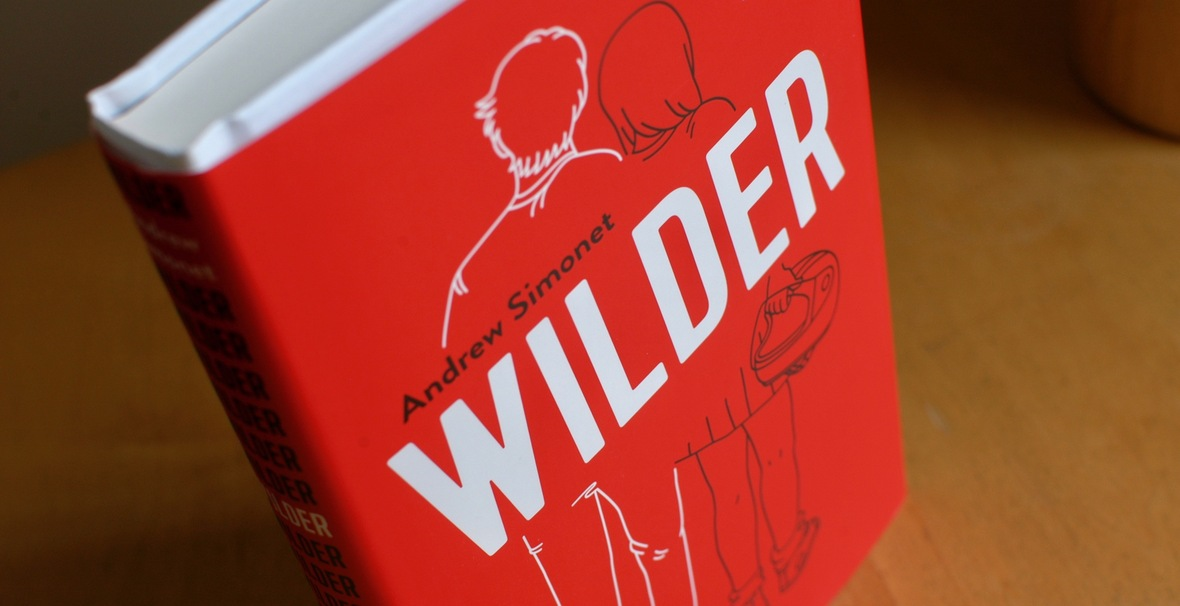# Some people have asked: Anything we can do to help Wilder?

Yes yes.

You can leave a rating or review on Amazon or on Goodreads. In this age of algorithms, these massively affect how people find books.

You can request Wilder at your local library or bookstore. Librarians and booksellers are an author's best allies. The more of them who know the book, the more readers will find it.

 table div table+table+table+table+table+table+table+table+table+table+table+table+table+table+table+table+table+table+table div table{width:100%;padding:0}table div table+table+table+table+table+table+table+table+table+table+table+table+table+table+table+table+table+table+table div table img{width:96.23%;padding:0;float:none}table div table+table+table+table+table+table+table+table+table+table+table+table+table+table+table+table+table+table+table div table td{width:100%;padding:0 1.88% 18px}/* styles */# After the book launch, I went to paradise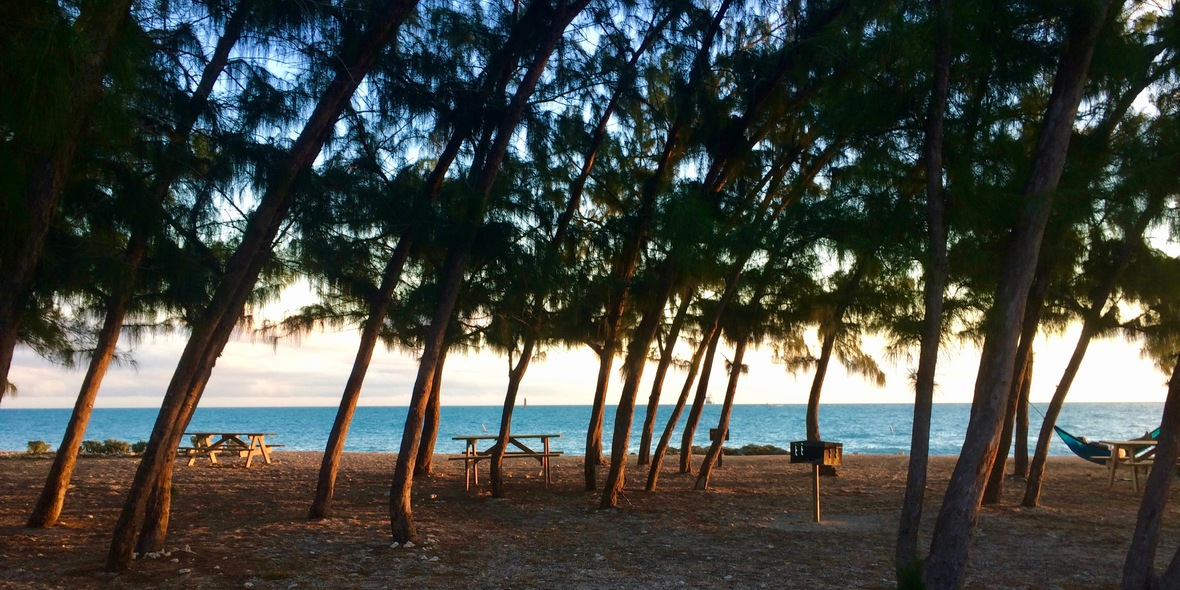/* styles */ The day after the Wilder launch party, I left for a fabulous residency at The Studios of Key West. Oh my goddess. All you artists should apply, next deadline: May 15.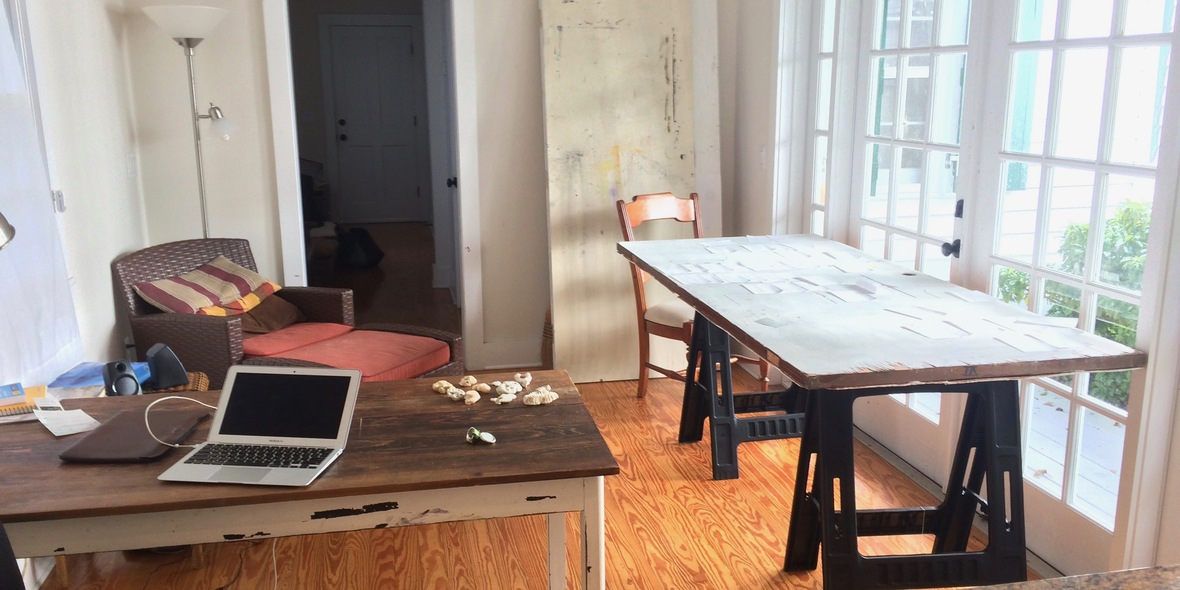This is my cottage where I worked on my next novel and ate pineapple.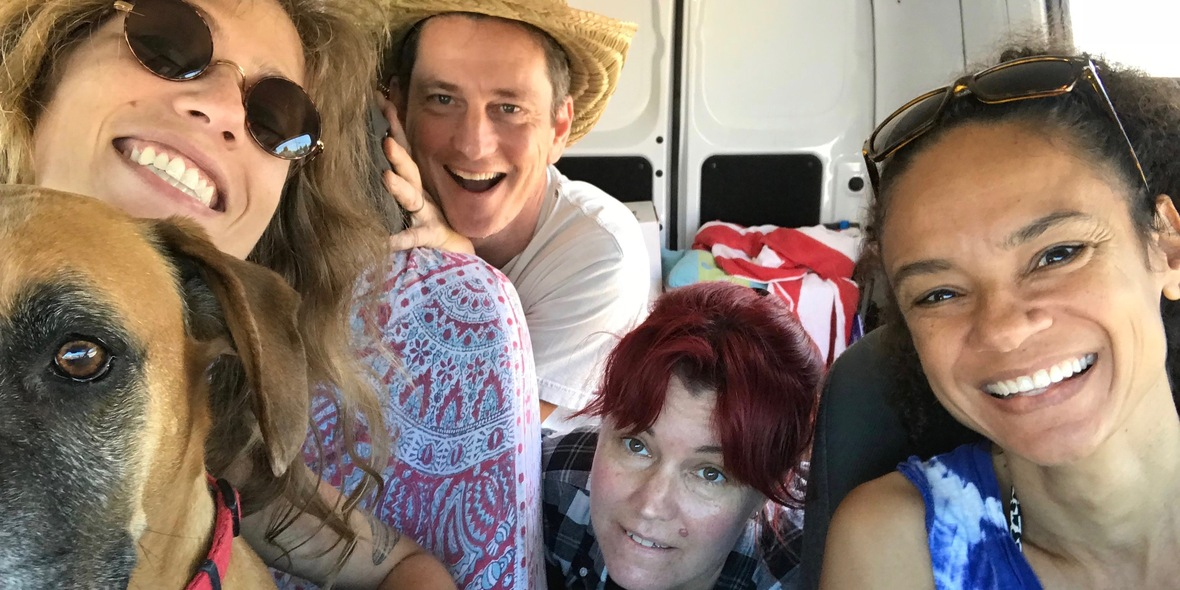/* styles */ Here are fellow artists-in-residents Beatriz Chachamovits (Brazil) and Isabelle Hayeur (Canada), and Sally Binard, Key West artist and community host.Sally took us with Captain Bill on an incredible boat trip through the mangrove islands.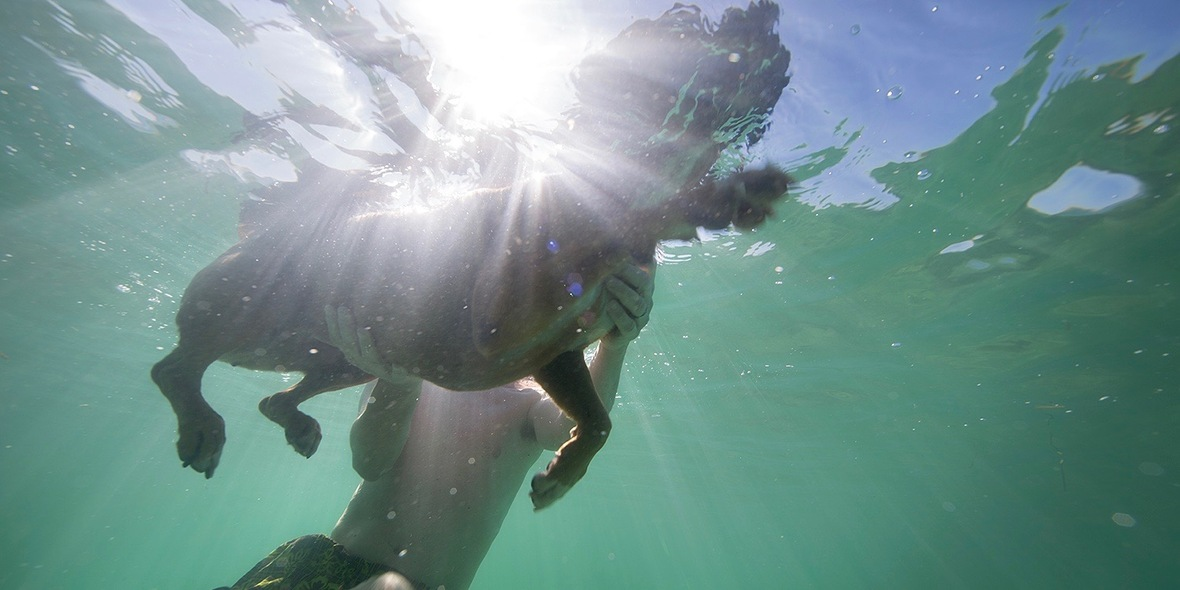I swam with Sally's dog.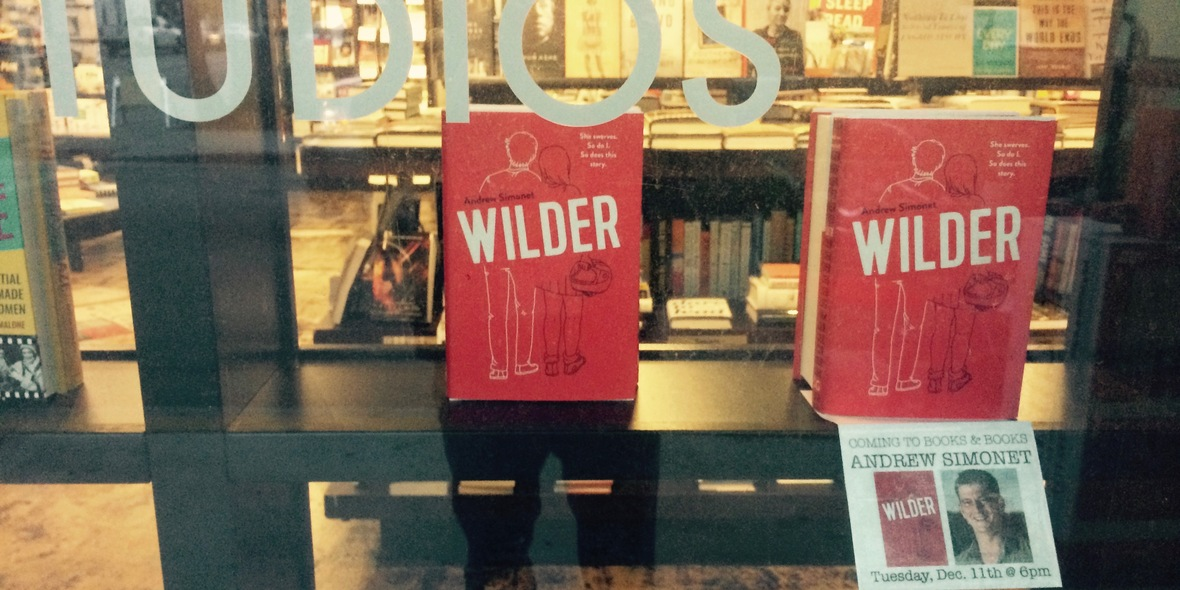I gave a reading at JUDY BLUME'S BOOKSTORE. Sigh. Judy was out of town. Still.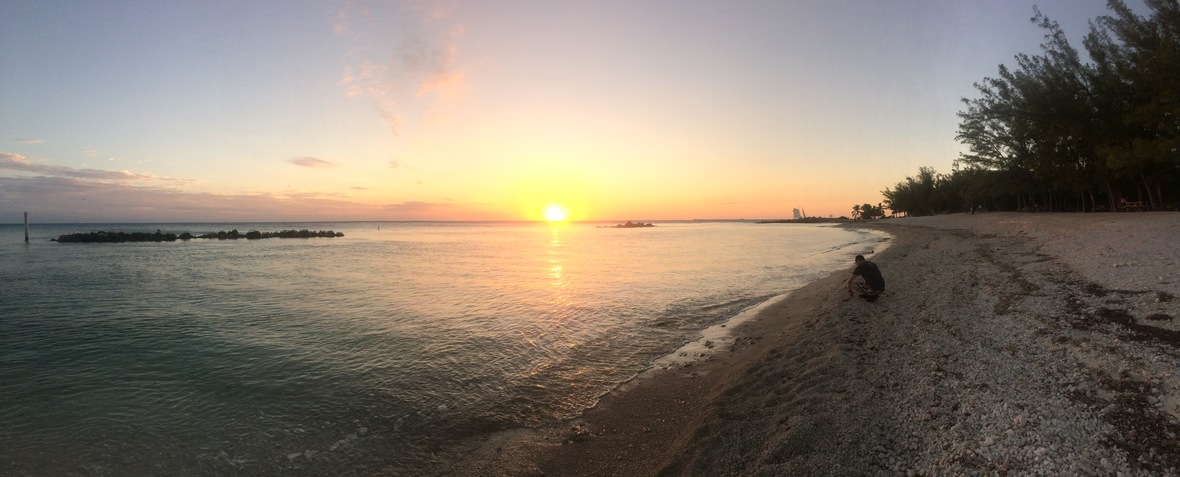And I swam every day at this beach. I tried to be in the water as the sun set. That is my definition of happiness.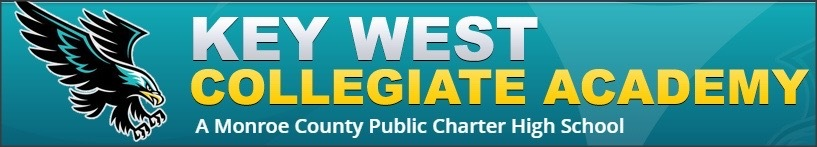# This is the view out their window:/* styles */ It’s a school for kids who, for a range of reasons, aren’t working out at the regular high school. My conversation when I arrived: PRINCIPAL: “So, it’s right after lunch, and it’s always hard to get the students to focus.” ME: “Totally. I understand.” PRINCIPAL: “And they might not look like they’re listening, but they are.” ME: “Oh. OK.” PRINCIPAL: “You know, they might have their eyes closed, or be reading a book, but they’re paying attention.” ME: “Reading a book?” PRINCIPAL: “Just know they’re really excited on the inside.” It turns out Wilder, with all its swears and fistfights and badassery, is a great fit for a high school of misfits. I had em right here.

# And I got a taste of the fabulous and fabulously odd Key West world. They are wildly into Christmas. This is the mayor’s house:# This quote from John Viele’s The Florida Keys says a lot:# I will miss Paddy Whack and Old Gilbert and all their guileless hearts. Thank you, Key West.

 table div table+table+table+table+table+table+table+table+table+table+table+table+table+table+table+table+table+table+table+table+table+table+table+table+table+table+table+table+table+table+table+table+table+table+table+table+table+table+table div table{width:100%;padding:0}table div table+table+table+table+table+table+table+table+table+table+table+table+table+table+table+table+table+table+table+table+table+table+table+table+table+table+table+table+table+table+table+table+table+table+table+table+table+table+table div table img{width:96.23%;padding:0;float:none}table div table+table+table+table+table+table+table+table+table+table+table+table+table+table+table+table+table+table+table+table+table+table+table+table+table+table+table+table+table+table+table+table+table+table+table+table+table+table+table div table td{width:100%;padding:0 1.88% 18px}/* styles */table div table+table+table+table+table+table+table+table+table+table+table+table+table+table+table+table+table+table+table+table+table+table+table+table+table+table+table+table+table+table+table+table+table+table+table+table+table+table+table+table div table td,table.module-39{width:100%;padding:0}table div table+table+table+table+table+table+table+table+table+table+table+table+table+table+table+table+table+table+table+table+table+table+table+table+table+table+table+table+table+table+table+table+table+table+table+table+table+table+table+table div table{width:100%;float:none;margin-left:auto;margin-right:auto;padding:0}table div table+table+table+table+table+table+table+table+table+table+table+table+table+table+table+table+table+table+table+table+table+table+table+table+table+table+table+table+table+table+table+table+table+table+table+table+table+table+table+table div table a{border:0 none;text-decoration:none}table div table+table+table+table+table+table+table+table+table+table+table+table+table+table+table+table+table+table+table+table+table+table+table+table+table+table+table+table+table+table+table+table+table+table+table+table+table+table+table+table div table img{width:100%!important;border:0 none;text-decoration:none}/* styles */